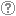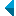# Search the repositoryQuery: search in TitleAuthorAbstractKeywordsFull textYear of publishing ANDORAND NOT search in TitleAuthorAbstractKeywordsFull textYear of publishing ANDORAND NOT search in TitleAuthorAbstractKeywordsFull textYear of publishing ANDORAND NOT search in TitleAuthorAbstractKeywordsFull textYear of publishing Work type: All work types Habilitation (m4) Specialist thesis (m3) High school thesis (m6) Bachelor work * (dip) Master disertations * (mag) Doctorate disertations * (dok) Research Data or Corpuses (data) * old and bologna study programme Language: All languagesSlovenianEnglishGermanCroatianSerbianBosnianBulgarianCzechFinnishFrenchGerman (Austria)HungarianItalianJapaneseLithuanianNorwegianPolishRussianSerbian (cyrillic)SlovakSpanishSwedishTurkishUnknown Search in: RUP    FAMNIT - Faculty of Mathematics, Science and Information Technologies    FHŠ - Faculty of Humanities    FM - Faculty of Management    FTŠ Turistica - Turistica – College of Tourism Portorož    FVZ - Faculty of Health Sciences    IAM - Andrej Marušič Institute    PEF - Faculty of Education    UPR - University of Primorska    ZUP - University of Primorska PressCOBISS    Fakulteta za humanistične študije, Koper    Fakulteta za management Koper in Pedagoška fakulteta Koper    Fakulteta za vede o zdravju, Izola    Knjižnica za tehniko, medicino in naravoslovje, Koper    Turistica, Portorož    Znanstveno-raziskovalno središče Koper Options: Show only hits with full text Reset

 1 - 10 / 3112341.The Terwilliger algebra of a distance-regular graph of negative typeŠtefko Miklavič, 2009, original scientific articleAbstract: Let ▫$\Gamma$▫ denote a distance-regular graph with diameter ▫$D \ge 3$▫. Assume ▫$\Gamma$▫ has classical parameters ▫$(D,b,\alpha,\beta)▫$ with ▫$b < -1$▫. Let ▫$X$▫ denote the vertex set of ▫$\Gamma$▫ and let ▫$A \in {\mathrm{Mat}}_X(\mathbb{C})$▫ denote the adjacency matrix of ▫$\Gamma$▫. Fix ▫$x \in X$▫ and let $A^\ast \in {\mathrm{Mat}}_X(\mathbb{C})$ denote the corresponding dual adjacency matrix. Let ▫$T$▫ denote the subalgebra of ${\mathrm{Mat}}_X(\mathbb{C})$ generated by ▫$A,A^\ast$▫. We call ▫$T$▫ the Terwilliger algebra of ▫$\Gamma$▫ with respect to ▫$x$▫. We show that up to isomorphism there exist exactly two irreducible ▫$T$▫-modules with endpoint 1; their dimensions are ▫$D$▫ and ▫$2D-2$▫. For these ▫$T$▫-modules we display a basis consisting of eigenvectors for ▫$A^\ast$▫, and for each basis we give the action of ▫$A$▫.Found in: ključnih besedahKeywords: distance-regular graph, negative type, Terwilliger algebraPublished: 15.10.2013; Views: 2728; Downloads: 105Full text (0,00 KB) 2.A complexitystudy of distance variants of covering and domination problems in H-free graphsMirza Krbezlija, 2021, master's thesisFound in: ključnih besedahSummary of found: ...distance-k vertex cover, distance k-edge cover, H-free graph, polynomial-time algorithm, NP-complete problem, dichotomy theorem...Keywords: distance-k dominating set, distance-k edge dominating set, distance-k vertex cover, distance k-edge cover, H-free graph, polynomial-time algorithm, NP-complete problem, dichotomy theoremPublished: 18.10.2021; Views: 451; Downloads: 15Full text (0,00 KB) 3.Distance-balanced graphs: Symmetry conditionsAleksander Malnič, Klavdija Kutnar, Dragan Marušič, Štefko Miklavič, 2006, original scientific articleAbstract: A graph ▫$X$▫ is said to be distance-balanced if for any edge ▫$uv$▫ of ▫$X$▫, the number of vertices closer to ▫$u$▫ than to ▫$v$▫ is equal to the number of vertices closer to ▫$v$▫ than to ▫$u$▫. A graph ▫$X$▫ is said to be strongly distance-balanced if for any edge ▫$uv$▫ of ▫$X$▫ and any integer ▫$k$▫, the number of vertices at distance ▫$k$▫ from ▫$u$▫ and at distance ▫$k+1$▫ from ▫$v$▫ is equal to the number of vertices at distance ▫$k+1$▫ from ▫$u$▫ and at distance ▫$k$▫ from ▫$v$▫. Exploring the connection between symmetry properties of graphs and the metric property of being (strongly) distance-balanced is the main theme of this article. That a vertex-transitive graph is necessarily strongly distance-balanced and thus also distance-balanced is an easy observation. With only a slight relaxation of the transitivity condition, the situation changes drastically: there are infinite families of semisymmetric graphs (that is, graphs which are edge-transitive, but not vertex-transitive) which are distance-balanced, but there are also infinite families of semisymmetric graphs which are not distance-balanced. Results on the distance-balanced property in product graphs prove helpful in obtaining these constructions. Finally, a complete classification of strongly distance-balanced graphs is given for the following infinite families of generalized Petersen graphs: GP▫$(n,2)$▫, GP▫$(5k+1,k)$▫, GP▫$(3k 3,k)$▫, and GP▫$(2k+2,k)$▫.Found in: ključnih besedahSummary of found: ...A graph ▫$X$▫ is said to be distance-balanced if...Keywords: graph theory, graph, distance-balanced graphs, vertex-transitive, semysimmetric, generalized Petersen graphPublished: 15.10.2013; Views: 3235; Downloads: 85Full text (0,00 KB) 4.Q-polynomial distance-regular graphs with a [sub] 1 [equal] 0 and a [sub] 2 [not equal] 0Štefko Miklavič, 2008, original scientific articleAbstract: Let ▫$\Gamma$▫ denote a ▫$Q$▫-polynomial distance-regular graph with diameter ▫$D \ge 3$▫ and intersection numbers ▫$a_1=0$▫, ▫$a_2 \ne 0$▫. Let ▫$X$▫ denote the vertex set of ▫$\Gamma$▫ and let ▫$A \in {\mathrm{Mat}}_X ({\mathbb{C}})$▫ denote the adjacency matrix of ▫$\Gamma$▫. Fix ▫$x \in X$▫ and let denote $A^\ast \in {\mathrm{Mat}}_X ({\mathbb{C}})$ the corresponding dual adjacency matrix. Let ▫$T$▫ denote the subalgebra of ▫$A{\mathrm{Mat}}_X ({\mathbb{C}})$▫ generated by ▫$A$▫, ▫$A^\ast$▫. We call ▫$T$▫ the Terwilliger algebra of ▫$\Gamma$▫ with respect to ▫$x$▫. We show that up to isomorphism there exists a unique irreducible ▫$T$▫-module ▫$W$▫ with endpoint 1. We show that ▫$W$▫ has dimension ▫$2D-2$▫. We display a basis for ▫$W$▫ which consists of eigenvectors for ▫$A^\ast$▫. We display the action of ▫$A$▫ on this basis. We show that ▫$W$▫ appears in the standard module of ▫$\Gamma$▫ with multiplicity ▫$k-1$▫, where ▫$k$▫ is the valency of ▫$\Gamma$▫.Found in: ključnih besedahSummary of found: ...Let ▫$\Gamma$▫ denote a ▫$Q$▫-polynomial distance-regular graph with diameter ▫$D \ge 3$▫ and intersection...Keywords: mathematics, graph theory, adjacency matrix, distance-regular graph, Terwilliger algebraPublished: 15.10.2013; Views: 3242; Downloads: 25Full text (0,00 KB) 5.Leonard triples and hypercubesŠtefko Miklavič, 2007, original scientific articleAbstract: Let ▫$V$▫ denote a vector space over ▫$\mathbb{C}$▫ with finite positive dimension. By a Leonard triple on ▫$V$▫ we mean an ordered triple of linear operators on ▫$V$▫ such that for each of these operators there exists a basis of ▫$V$▫ with respect to which the matrix representing that operator is diagonal and the matrices representing the other two operators are irreducible tridiagonal. Let ▫$D$▫ denote a positive integer and let ▫${\mathcal{Q}}_D$▫ denote the graph of the ▫$D$▫-dimensional hypercube. Let ▫$X$ denote the vertex set of ▫${\mathcal{Q}}_D$▫ and let ▫$A \in {\mathrm{Mat}}_X ({\mathbb{C}})$▫ denote the adjacency matrix of ▫${\mathcal{Q}}_D$▫. Fix ▫$x \in X$▫ and let ▫$A^\ast \in {\mathrm{Mat}}_X({\mathbb{C}})$▫ denote the corresponding dual adjacency matrix. Let ▫$T$▫ denote the subalgebra of ▫${\mathrm{Mat}}_X({\mathbb{C}})$ generated by ▫$A,A^\ast$▫. We refer to ▫$T$▫ as the Terwilliger algebra of ▫${\mathcal{Q}}_D$▫ with respect to ▫$x$▫. The matrices ▫$A$▫ and ▫$A^\ast$▫ are related by the fact that ▫$2iA = A^\ast A^\varepsilon - A^\varepsilon A^\ast$▫ and ▫$2iA^\ast = A^\varepsilon A - AA^\varepsilon$▫, where ▫$2iA^\varepsilon = AA^\ast - A^\ast A$▫ and ▫$i^2 = -1$▫. We show that the triple ▫$A$▫, ▫$A^\ast$▫, ▫$A^\varepsilon$▫ acts on each irreducible ▫$T$▫-module as a Leonard triple. We give a detailed description of these Leonard triples.Found in: ključnih besedahSummary of found: ...mathematics, graph theory, Leonard triple, distance-regular graph, hypercube, Terwilliger algebra...Keywords: mathematics, graph theory, Leonard triple, distance-regular graph, hypercube, Terwilliger algebraPublished: 15.10.2013; Views: 2914; Downloads: 115Full text (0,00 KB) 6.On the connectivity of bipartite distance-balanced graphsŠtefko Miklavič, Primož Šparl, 2012, original scientific articleAbstract: A connected graph ▫$\varGamma$▫ is said to be distance-balanced whenever for any pair of adjacent vertices ▫$u,v$▫ of ▫$\varGamma$▫ the number of vertices closer to ▫$u$▫ than to ▫$v$▫ is equal to the number of vertices closer to ▫$v$▫ than to ▫$u$▫. In [K. Handa, Bipartite graphs with balanced ▫$(a,b)$▫-partitions, Ars Combin. 51 (1999), 113-119] Handa asked whether every bipartite distance-balanced graph, that is not a cycle, is 3-connected. In this paper the Handa question is answered in the negative. Moreover, we show that a minimal bipartite distance-balanced graph, that is not a cycle and is not 3-connected, has 18 vertices and is unique. In addition, we give a complete classification of non-3-connected bipartite distance-balanced graphs for which the minimal distance between two vertices in a 2-cut is three. All such graphs are regular and for each ▫$k \geq 3$▫ there exists an infinite family of such graphs which are ▫$k$▫-regular.Furthermore, we determine a number of structural properties that a bipartite distance-balanced graph, which is not 3-connected, must have. As an application, we give a positive answer to the Handa question for the subfamily of bipartite strongly distance-balanced graphs.Found in: ključnih besedahSummary of found: ...A connected graph ▫$\varGamma$▫ is said to be distance-balanced whenever...Keywords: graph theory, connected graphs, connectivity, distance-balanced graphs, bipartite graphsPublished: 15.10.2013; Views: 2560; Downloads: 92Full text (0,00 KB) 7.Large sets of long distance equienergetic graphsDragan Stevanović, 2009, original scientific articleAbstract: Distance energy of a graph is a recent energy-type invariant, defined as the absolute deviation of the eigenvalues of the distance matrix of the graph. Two graphs of the same order are said to be distance equienergetic if they have equal distance energy, while they have distinct spectra of their distance matrices. Examples of pairs of distance equienergetic graphs appear in the literature already, but most of them have diameter two only. We describe here the distance spectrum of a special composition of regular graphs, and, as an application, we show that for any ▫$n \ge 3$▫, there exists a set of ▫$n + 1$▫ distance equienergetic graphs which have order ▫$6n$▫ and diameter ▫$n - 1$▫ each.Found in: ključnih besedahSummary of found: ...Distance energy of a graph is a recent energy-type invariant, defined as...Keywords: graph theory, distance spectrum, distance energy, join, regular graphsPublished: 15.10.2013; Views: 2760; Downloads: 134This document has more files! More... 8.The strongly distance-balanced property of the generalized Petersen graphsŠtefko Miklavič, Dragan Marušič, Aleksander Malnič, Klavdija Kutnar, 2009, original scientific articleAbstract: A graph ▫$X$▫ is said to be strongly distance-balanced whenever for any edge ▫$uv$▫ of ▫$X$▫ and any positive integer ▫$i$▫, the number of vertices at distance ▫$i$▫ from ▫$u$▫ and at distance ▫$i + 1$▫ from ▫$v$▫ is equal to the number of vertices at distance ▫$i + 1$▫ from ▫$u$▫ and at distance ▫$i$▫ from ▫$v$▫. It is proven that for any integers ▫$k \ge 2$▫ and ▫$n \ge k^2 + 4k + 1$▫, the generalized Petersen graph GP▫$(n, k)$▫ is not strongly distance-balanced.Found in: ključnih besedahSummary of found: ...A graph ▫$X$▫ is said to be strongly distance-balanced...Keywords: graph, strongy distance-balanced, generalized Petersen graphPublished: 15.10.2013; Views: 2423; Downloads: 125This document has more files! More... 9.On bipartite graphs with exactly one irreducible T-module with endpoint 1, which is thinŠtefko Miklavič, Blas Fernández, 2021, original scientific articleFound in: ključnih besedahSummary of found: ...distance-biregular graph, Terwilliger algebra, irreducible module...Keywords: distance-biregular graph, Terwilliger algebra, irreducible modulePublished: 18.10.2021; Views: 272; Downloads: 23Full text (0,00 KB) 10.Classification of tetravalent distance magic circulant graphsŠtefko Miklavič, Primož Šparl, 2021, original scientific articleFound in: ključnih besedahSummary of found: ...distance magic labeling, distance magic graph, circulant graph...Keywords: distance magic labeling, distance magic graph, circulant graphPublished: 18.10.2021; Views: 283; Downloads: 19Full text (0,00 KB)
Search done in 0 sec.